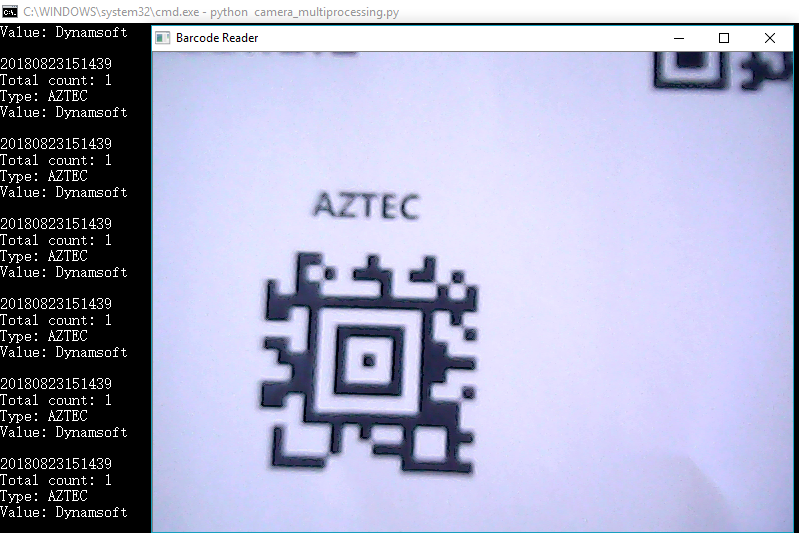×

# How to Use Multiprocessing to Optimize Python Barcode Reader

Previously, I demonstrated how to use OpenCV and Dynamsoft Barcode SDK to build a Python barcode reader. There’s one problem that when barcode decoding takes a long time, the webcam video didn’t play smoothly. I also tried multithread but failed to tackle the issue either due to the Python GIL(Global Interpreter Lock). Now I’m inspired by multiprocessing which is the recommended way to break through the bottleneck.

## Multiprocessing for Python Barcode Reader

Create a process for decoding barcodes and two queues for sharing data.

``````from multiprocessing import Process, Queue
frame_queue = Queue(4)
finish_queue = Queue(1)
dbr_proc = Process(target=dbr_run, args=(
frame_queue, finish_queue))
dbr_proc.start()

``````

Start video capture and keep adding video frames to frame_queue.

``````    vc = cv2.VideoCapture(0)

if vc.isOpened():  # try to get the first frame
else:
return

base = 2
count = 0
while True:
cv2.imshow(windowName, frame)

count %= base
if count == 0:
try:
frame_queue.put_nowait(frame)
except:
try:
while True:
frame_queue.get_nowait()
except:
pass

count += 1

``````

To share video frames to barcode decoding process, we can use either Queue.put() or Queue.put_nowait(). The difference is the put() method will block until a free slot is available. If the barcode process takes a long time for decoding one frame, the queue will be full quickly. To make camera video play smoothly, we should use the non-blocked method put_nowait(). If the queue is full, it will raise an exception in which we can drop the old frames in the queue. We have to keep video frames up to date.

In the barcode decoding process, we continuously fetch frames from the queue.

``````def dbr_run(frame_queue, finish_queue):
while finish_queue.qsize() == 0:
try:
inputframe = frame_queue.get_nowait()
results = dbr.decodeBuffer(inputframe, config.barcodeTypes)
if (len(results) > 0):
print(get_time())
print("Total count: " + str(len(results)))
for result in results:
print("Type: " + result)
print("Value: " + result + "\n")
except:
pass

dbr.destroy()

``````

So far, the code can work. However, if you run the code and try to quit the app, you may see the following error message.

``````Traceback (most recent call last):

File "E:\Programs\Python\Python36\lib\multiprocessing\queues.py", line 236, in _feed

send_bytes(obj)

File "E:\Programs\Python\Python36\lib\multiprocessing\connection.py", line 200, in send_bytes

self._send_bytes(m[offset:offset + size])

File "E:\Programs\Python\Python36\lib\multiprocessing\connection.py", line 290, in _send_bytes

nwritten, err = ov.GetOverlappedResult(True)

BrokenPipeError: [WinError 109] The pipe has been ended
``````

One more thing we have to do is to clear and close queues before exiting the app.

``````def clear_queue(queue):
try:
while True:
queue.get_nowait()
except:
pass
queue.close()

``````

If you don’t remove all items, you will still see the error even if you close the queue.

Here is the final look of my Python barcode reader:## Source Code

https://github.com/dynamsoft-dbr/python/blob/master/examples/camera/camera_multiprocessing.py